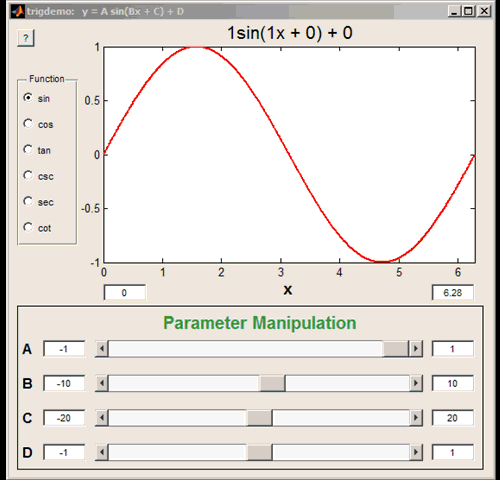# Exploring Trigonometric Functions

Brett's Pick this week is TrigDemo, by Matt Fig.

Matt is one of the more prolific (and popular) authors on the File Exchange; his 36 submissions (to date) have been downloaded a total of 1741 times in the past 30 days. Today's Pick is a very simple, very cool tool for exploring trigonometric functions. With an easy-to-use GUI, one can plot any equation of the form y(x) = A trig(Bx + C) + D, where "trig" can be any one of: {'sine', 'cosine', 'tangent', 'cosecant', 'secant', 'cotangent'}. Then, with easy-to-use radio buttons and sliders, one can interactively change that trigonometric function, or see the effects of changing any of the variables A, B, C, or D.I like to think that MATLAB has a role in teaching mathematics at a variety of levels. Matt's GUI helps trigonometry students visualize and master the fundamentals (and provides a refresher for those of us who took trigonometry longer ago than we care to admit!) Simply, a very nice utility.

Take TrigDemo for a test spin, and let us know what you think, or leave a comment for Matt here.

Published with MATLAB® 7.11

|

### コメント

コメントを残すには、ここ をクリックして MathWorks アカウントにサインインするか新しい MathWorks アカウントを作成します。